# Subtracting Positive and Negative Numbers

Subtracting positive numbers, such as 4 - 2, is easy. When we subtract negative numbers or subtract negative numbers to positive numbers, it gets more complicated.
Here are some simple rules to follow when subtracting negative numbers.

## Rule 1: Subtracting a positive number from a positive number – it’s just normal subtraction.

For example: this is what you have learned before. 6 – 3 are two positive numbers. So solve this equation the way you always have: 6 – 3 = 3.

## Rule 2: Subtracting a positive number from a negative number – start at the negative number and count backwards.

For example: Say, we have the problem -2 – 3. Using the number line, let’s start at -2.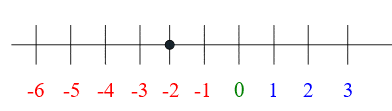Now count backwards 3 units. So keep counting back three spaces from -2 on the number line.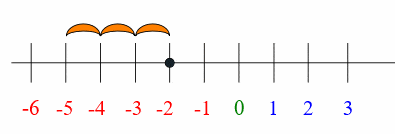The answer is -2 – 3 = -5.

## Rule 3: Subtracting a negative number from a negative number – a minus sign followed by a negative sign, turns the two signs into a plus sign.

So, instead of subtracting a negative, you are adding a positive. Basically, - (-4) becomes +4, and then you add the numbers.

For example, say we have the problem -2 - –4. This would read “negative two minus negative 4”. So we’re changing the two negative signs into a positive, so the equation now becomes -2 + 4.

On the number line, it starts at -2.Then we move forward 4 units: +4.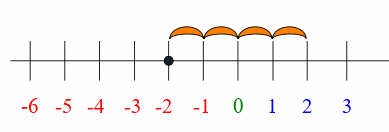class="green-text">The answer is -2 – (-4) = 2.

## Rule 4: Subtracting a negative number from a positive number – turn the subtraction sign followed by a negative sign into a plus sign.

So, instead of subtracting a negative, you’re adding a positive. So the equation turns into a simple addition problem.

For example: let’s say we have the problem 2 – (-3). This reads “two minus negative three”. The – (-3) turns into +3.

On the number line we start at 2.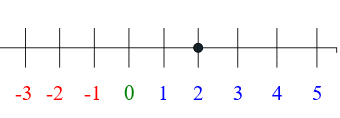Then we move forward three units: 2 + 3.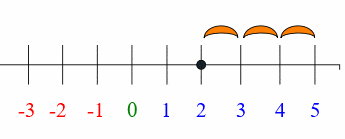The answer is 2 – (-3) = 5.Become a Member

This content is available to members only.